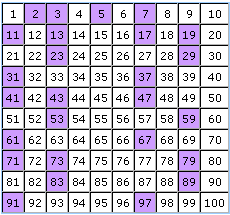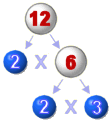Go to contentA prime number is a number with exactly two factors, itself and 1. Almost all prime numbers are also odd. The exception is 2 which is even but also a prime number.

The table to the left highlights all the prime numbers between 1 and 100.

Any number can broken down into its prime factors. A good way to do this is to make a factor tree, then the number can be written as a prime factorisation, for example:Click for slideshow

Summary/BackgroundA factor is a whole number that divides exactly into another number. A prime number is a number with only two factors: itself and 1. The first prime numbers are 2, 3, 5, 7, 11, 13...
The diagram on the right is a factor tree, showing the prime factors of 12 to be 2 and 3. Notice that 12 = 2 x 2 x 3. In this way 12 has been expressed as a product of its prime factors.You can get a better display of the maths by downloading special TeX fonts from jsMath. In the meantime, we will do the best we can with the fonts you have, but it may not be pretty and some equations may not be rendered correctly.

Glossary

factorisation

The process of expressing a term as a product of factors.

function

A rule that connects one value in one set with one and only one value in another set.

prime

a positive integer which is divisible only by itself and 1

speed

velocity without direction; a scalar quantity

tree

A connected graph with no cycles.

union

The union of two sets A and B is the set containing all the elements of A and B.

Full Glossary List

This question appears in the following syllabi:

SyllabusModuleSectionTopicExam Year
AQA GCSE (9-1) Foundation (UK)N: Structure and CalculationN4: Factors and MultiplesPrime Numbers-
CBSE X (India)Number SystemsReal NumbersFundamental theorem of arithmetic-
CIE IGCSE (9-1) Maths (0626 UK)1 NumberB1.1 Understanding NumbersPrime Numbers-
Edexcel GCSE (9-1) Foundation (UK)N: Structure and CalculationN4: Factors and MultiplesPrime Numbers-
GCSE Foundation (UK)NumberArithmeticPrime numbers-
OCR GCSE (9-1) Foundation (UK)1: Number Operations and Integers1.02b: Prime NumbersPrime Numbers-
Universal (all site questions)AArithmeticPrime numbers-